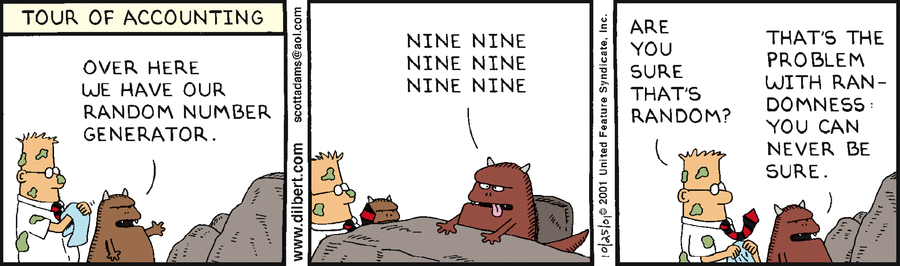# Statistics - Random Walk

## Walk

A random walk is a mathematical formalization of a path that consists of a succession of random steps. For example, the price of a fluctuating stock can be modelled as random walks. Half the time it goes up, half the time it goes down.

## Example

``````width=600;
height=300;

randomWalk = ( function() {
let y = height/2, values = [y];
for (let x = 0; x < width; ++x) values.push(y = y + (Math.random() - 0.5) * 40 + (height/2 - y) * 0.1);
return values;
})()

canvas = document.getElementById("graph");
context = canvas.getContext("2d");
context.beginPath();
context.moveTo(0, randomWalk);
for (let x = 0; x < width; ++x) context.lineTo(x, randomWalk[x]);
context.lineJoin = context.lineCap = "round";
context.strokeStyle = "black";
context.stroke();```
```
````<canvas id="graph" width="600" height="300"></canvas>`
```

Recommended PagesNumber - Random (Stochastic|Independent) or (Balanced)

Think of randomness as a lack of pattern. Something random should be unpredictable. We shouldn’t be able to predict the next value of the sequence The degree to which a system has no pattern is known...R - Linear Discriminant Analysis (LDA)

Linear Discriminant Analysis in R Fit the model Print it by tapping its name where: the prior probabilities are just the proportions of false and true in the data set. It's kind of a random...